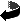# Pseudodifferential operators on manifolds with a Lie structure at infinity by Bernd Ammann, Robert Lauter, Victor Nistor

Pseudodifferential operators on manifolds with a Lie structure at infinity (.dvi, .ps,.ps.gz oder .pdf)
Ann. of Math. 165, 717-747 (2007)

### Abstract

Several interesting examples of non-compact manifolds $M_0$ whose geometry at infinity is described by Lie algebras of vector fields $\VV \subset \Gamma(M; TM)$ (on a compactification of $M_0$ to a manifold with corners $M$) were studied in \cite{meicm, MelroseScattering, VasyN}. In \cite{aln1}, the geometry of manifolds described by Lie algebras of vector fields -- baptised manifolds with a Lie structure at infinity'' there -- was studied from an axiomatic point of view. In this paper, we define and study the algebra $\Psi_{1,0,\VV}^\infty(M_0)$, which is an algebra of pseudodifferential operators canonically associated to a manifold $M_0$ with the Lie structure at infinity $\VV \subset \Gamma(M;TM)$. We show that many of the properties of the usual algebra of pseudodifferential operators on a compact manifold extend to $\Psi_{1,0,\VV}^\infty(M_0)$. We also consider the algebra $\DiffV{*}(M_0)$ of differential operators on $M_0$ generated by $\VV$ and $\CI(M)$, and show that $\Psi_{1,0,\VV}^\infty(M_0)$ is a microlocalization'' of $\DiffV{*}(M_0)$. We also define semi-classical and suspended'' versions of the algebra $\Psi_{1,0,\VV}^\infty(M_0)$. Thus our constructions solves a conjecture of Melrose \cite{meicm}.Zurück zur Homepage

Bernd Ammann, Robert Lauter, Victor Nistor,
The Paper was written on 19.02.2003
Last update 7.1.2010